# Repeated Addition - Definition with Examples

The Complete K-5 Math Learning Program Built for Your Child

• 30 Million Kids

Loved by kids and parent worldwide

• 50,000 Schools

Trusted by teachers across schools

• Comprehensive Curriculum

Aligned to Common Core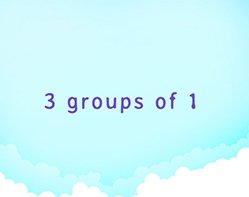Relate multiplication and addition; multiplying numbers can be thought of as adding a number to itself repeatedly.

Covers Common Core Curriculum 3.OA.1

Repeated addition is adding equal groups together. It is also known as multiplication. If the same number is repeated then, we can write that in the form of multiplication.

For example: 2 + 2 + 2 + 2 + 2

Here 2 is repeated 5 times, we can write this addition as 5 × 2.

Similarly, to solve a multiplication problem through repeated addition, we repetitively group and add the same number again and again to find the answer.

Here are a few examples of repeated addition.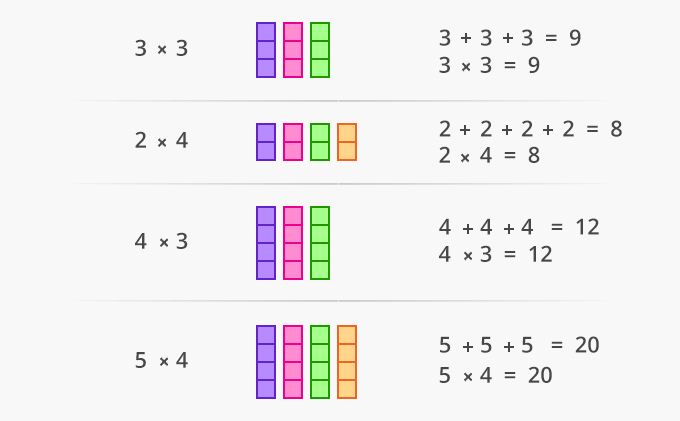Here’s another example of repeated addition used for multiplication in word problems.

There are 5 groups of chickens. Each group has 3 chickens. How many chickens are there in all?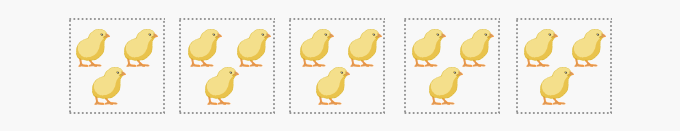There are 5 groups.

There are 3 chickens in each group.

 Add to find the total chickens.  3 + 3 + 3 + 3 + 3 = 15 Multiply to find the  total chickens.  5 × 3 = 15

There are 15 chickens in all.

As multiplication is repeated addition, each repeated addition can be written in two ways:

For example: 6 + 6 + 6 + 6 = 24 can be written as 4 × 6 = 24 and also as 6 × 4 = 24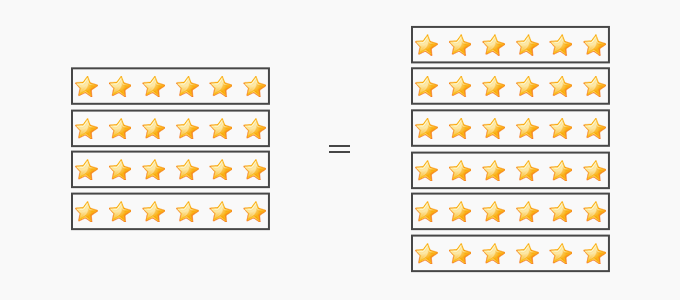6 + 6 + 6 + 6 = 24 4 × 6 = 24 4 + 4 + 4 + 4 + 4 + 4 = 24 6 × 4 = 24

Repeated addition is also helpful in learning multiplication facts. For example if you do not know the 7× 3 facts yet, you may find it easier to work out 7 × 3 by writing 3 + 3 + 3 + 3 + 3 + 3 + 3 or 7 + 7 + 7; and then slowly adding.  It may also be helpful with larger numbers, such as 5 × 40. It is more comfortable writing 40 + 40 + 40 + 40 + 40 and then adding on the tens.

 Fun Facts On a number line, one can skip count to add repeatedly to multiply.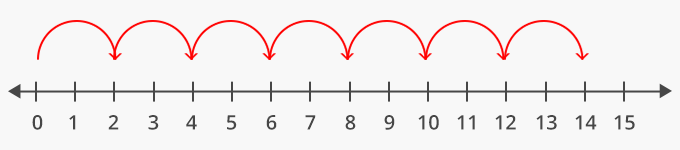Won Numerous Awards & Honors